3.NF.2.A.-Test1C Represent a fraction on a number line
 Name:    3.NF.2.A.-Test1C Represent a fraction on a number line

Multiple Choice
Identify the choice that best completes the statement or answers the question.

1.

At which dot would the fraction 3/6 be placed on the following number line?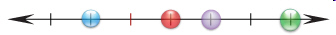a. green c. purple b. blue d. red

2.

What fraction is represented by the red dot on the number line?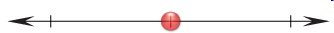a. 1/2 c. 1/3 b. 2/3 d. 1/4

3.

At which dot would the fraction 3/3 be placed on the following number line?a. green c. purple b. blue d. red

4.

At which dot would the number 2/3 be placed on the following number line?a. green c. purple b. blue d. red

5.

At which dot would the number 0/3 be placed on the following number line?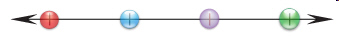a. green c. purple b. blue d. red

6.

At which dot would the number1/3 be placed on the following number line?a. green c. purple b. blue d. red

7.

At which dot would the number 6/6 be placed on the following number line?a. green c. purple b. blue d. red

8.

At which dot would the number 4/6 be placed on the following number line?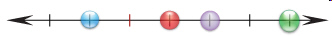a. green c. purple b. blue d. red

9.

At which dot would the number 1/6 be placed on the following number line?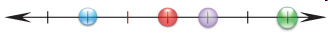a. green c. purple b. blue d. red

10.

What fraction is represented by the red dot on the number line?a. 3/6 c. 4/6 b. 2/6 d. 1/6